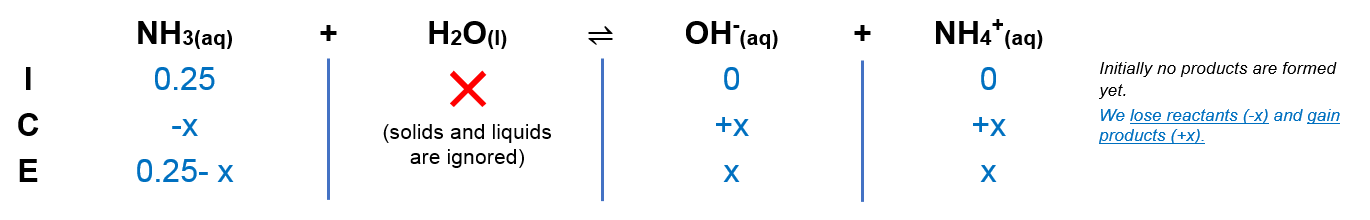# Problem: What is the pH of a solution in which 0.250 mol of NH3 (Kb = 1.8 × 10-5) is dissolved in sufficient water to make 1.00 L of solution?a. 2.67b. 11.33c. 4.93d. 13.4e. 9.07

###### FREE Expert Solution

We are being asked to calculate the pH for a 0.250 mol NH3 in 1 L solution

NH3 is a neutral amine, therefore we know that it is a weak base.

NH3(aq) + H2O(aq)  OH(aq) + NH4+(aq)Kb = 1.8 × 10-5

Calculate the molarity:

Molarity =  0.250 M

From this, we can construct an ICE table. Remember that liquids are ignored in the ICE table.The Kb expression for NH3 is:

Note that each concentration is raised by the stoichiometric coefficient: [NH3], [OH] and [NH4+] are raised to 1.

82% (100 ratings)###### Problem Details

What is the pH of a solution in which 0.250 mol of NH3 (Kb = 1.8 × 10-5) is dissolved in sufficient water to make 1.00 L of solution?

a. 2.67

b. 11.33

c. 4.93

d. 13.4

e. 9.07